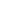Maths Tutoring - Year 2 St George & Sutherland Community College

# Maths Tutoring - Year 2

This practical course is designed to teach the student to master Stage 1 Mathematical concepts. This program integrates the components of working mathematically through whole number, addition and subtraction, multiplication and division, fractions and decimals, patterns and algebra.

Math coaching is individualised to cater for the educational needs of the students who wish to understand the key concepts in Math or for those looking for extension. Professional qualified and experienced teachers help your child realise their full potential through sequential instruction in small class settings.

The students will explore and strengthen any individual weaknesses they may be experiencing and consolidate the mathematical concepts needed to become proficient and confident mathematical users.

Through coaching the students have the opportunity to be taught how to use known facts, objects, diagrams and technology to explore mathematical problems and develop mathematical fluency. The students are shown how to link mathematical ideas and use appropriate language and diagrams to explain the strategies they used.

This coaching covers the correct use of mathematical terminology and some conventions allowing the student to use mental and written strategies to solve word problems while effectively using the 4 operations (addition, subtraction, multiplication & division).

The intended learning outcome is for the student to develop their skills in one or more of the following areas:

• count order, read & write two- and 3-digit numbers
• use mental strategies and concrete materials to add, subtract, multiply and divide, and solve problems.
• model 3 dimensional objects and two-dimensional shapes
• work with halves, quarters and eights
• relate addition and subtraction facts for sums to at least 20.
• tell the time on the hour, half and quarter-hour
• explore mathematical problems and develop mathematical fluency.

#### Entry Requirements:

• Student currently in Year 2

#### Course Materials:

Bring pen, pencils and paper Homework is given upon request.

If you wish to discuss your child’s individual needs or payment plan options please contact one of our educational specialists on 02 9580 7885 (Monday to Thursday 10am – 1 pm). For after-hours please leave a voice mail and you will be contacted the next working day.

Searching for an education program designed to enhance the student’s learning can be a confusing endeavor. Our FAQs are designed to help simplify this task.

\$250 \$125 Limited GST free
Maths Tutoring - Year 2

<p>This practical course is designed to teach the student to master Stage 1 Mathematical concepts. This program integrates the components of working mathematically through whole number, addition and

...
Mo Tu We Th Fr Sa Su\$250 \$125 Limited GST free
Maths Tutoring - Year 2

<p>This practical course is designed to teach the student to master Stage 1 Mathematical concepts. This program integrates the components of working mathematically through whole number, addition and

...
Mo Tu We Th Fr Sa Su